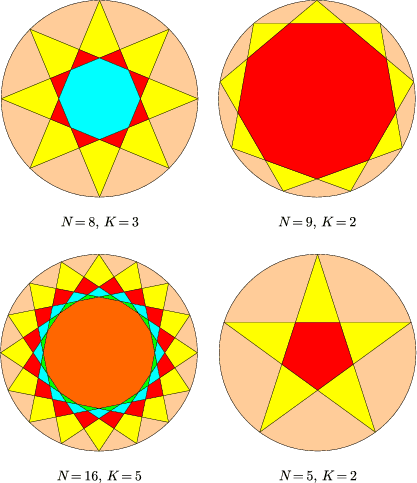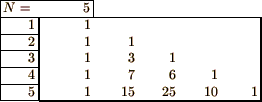Mathematical and Physical Journal
for High Schools
Issued by the MATFUND Foundation
 Already signed up? New to KöMaL?# Exercises and problems in InformaticsMarch 2003

I. 46. Suppose we are given the set H={1,2,...,N} (1$\displaystyle \le$N$\displaystyle \le$1000) and two of its arbitrary subsets A,B $\displaystyle \subset$H both having exactly K (1$\displaystyle \le$K$\displaystyle \le$N-1) elements. We say that the set A is less than the set B, if the maximal element of $\displaystyle A\setminus B$ is less than that of $\displaystyle B\setminus A$. Write a program (i46.pas, ...) which reads the values of N, K and L, then gives the Lth largest subset of H with K elements.

Example: if N=5 and K=2, then the L=1st subset is {5,4}, L=2nd subset is {5,3}, L=5th subset is {4,3}, L=10th subset is {2,1}. (10 points)

I. 47. Prepare a program (i47.pas, ...) which first displays a circle together with a regular N-gon (3$\displaystyle \le$N$\displaystyle \le$50) on its circumference (with one vertex at the top of the circle), then connects this topmost point with the Kth (1$\displaystyle \le$K$\displaystyle \le$N/2) vertex counterclockwise, then that one with the 2Kth vertex and so on, until we get back to the original point. The program should finally colour the figure in such a way that closed regions of similar type have the same, while those of different type have different colours, see the Figures.
(10 points)I. 48. There is a class of N (1$\displaystyle \le$N$\displaystyle \le$20) students. We are interested in the number of ways of dividing them into K non-empty groups.

If N is entered, your sheet (i48.xls) should compute the number of possible ways a class of I (I=1,2,...,N) students can be divided into K non-empty groups, then display the result in the Ith row and Kth column of the framed area in the Figure. If the corresponding field makes no sense, then no value should be displayed in that position.
(10 points)